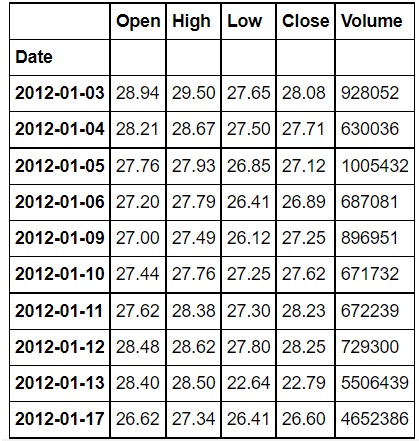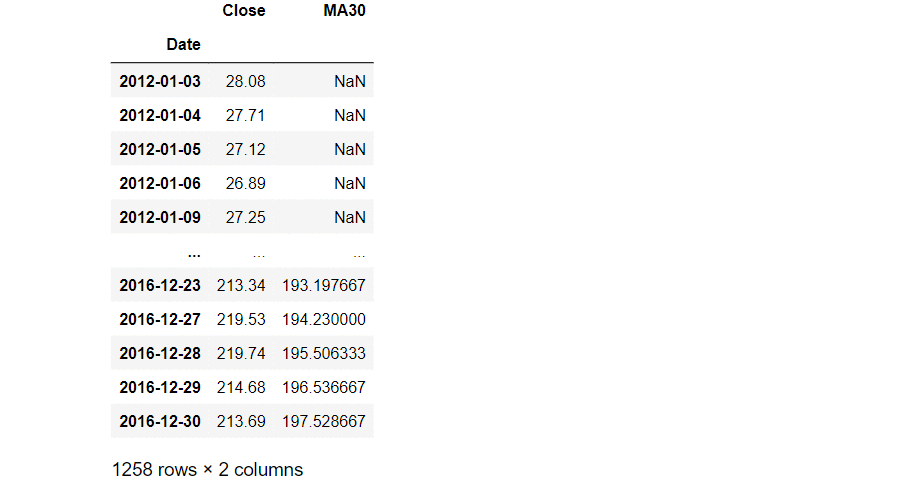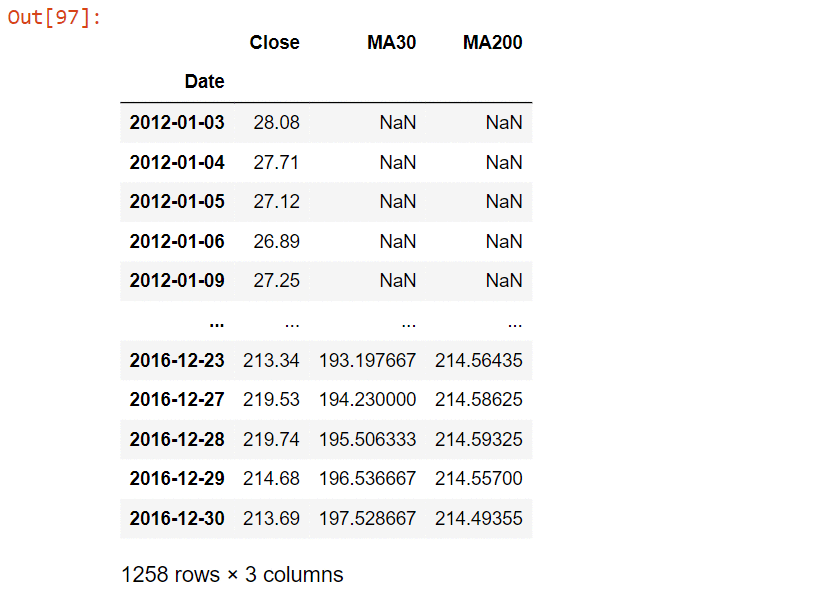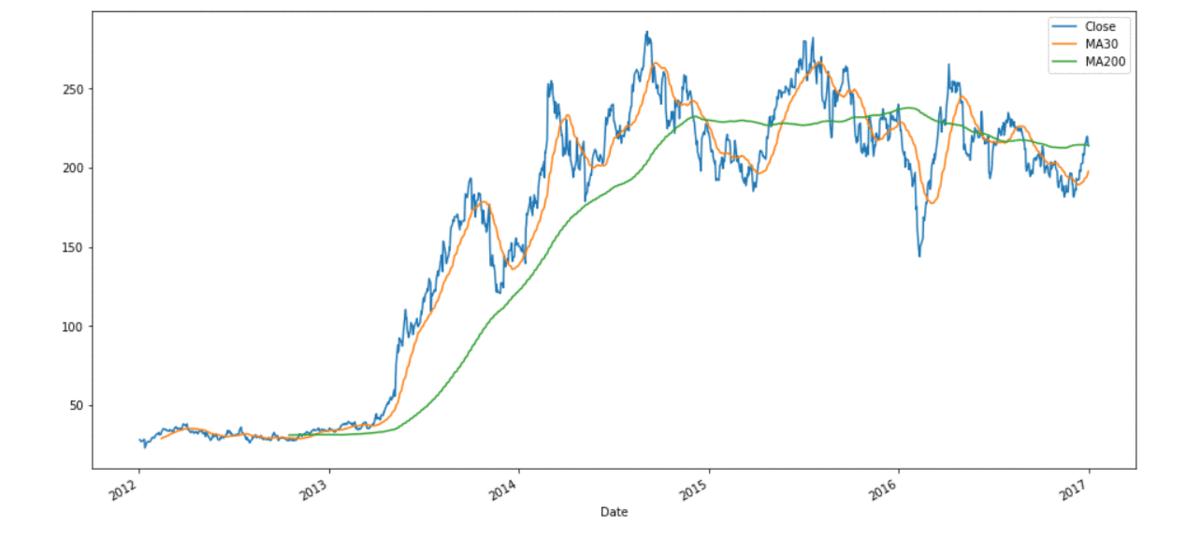# Pandas – Rolling mean by time interval

• Difficulty Level : Hard
• Last Updated : 24 Oct, 2021

In this article, we will be looking at how to calculate the rolling mean of a dataframe by time interval using Pandas in Python.

Pandas dataframe.rolling() is a function that helps us to make calculations on a rolling window. In other words, we take a window of a fixed size and perform some mathematical calculations on it.

Attention geek! Strengthen your foundations with the Python Programming Foundation Course and learn the basics.

To begin with, your interview preparations Enhance your Data Structures concepts with the Python DS Course. And to begin with your Machine Learning Journey, join the Machine Learning - Basic Level Course

Syntax: DataFrame.rolling(window, min_periods=None, center=False, win_type=None, on=None, axis=0).mean()

Parameters :

• window : Size of the window. That is how many observations we have to take for the calculation of each window.
• min_periods : Least number of observations in a window required to have a value (otherwise result is NA).
• center : It is used to set the labels at the center of the window.
• win_type : It is used to set the window type.
• on : Datetime column of our dataframe on which we have to calculate rolling mean.
• axis : integer or string, default 0

Dataset Used: Tesla_Stock

## Python3

 `# import pandas as pd``import` `pandas as pd`

## Python3

 `# importing Data``tesla_df ``=` `pd.read_csv(``'Tesla_Stock.csv'``, index_col``=``'Date'``, ``                       ``parse_dates``=``True``)`` ` `# printing the dataFrame``tesla_df.head(``10``)`

Output:We will be calculating the rolling mean of the column ‘Close’ of the DataFrame.

## Python3

 `# Updating the dataFrame with just the ``# column 'Close' as others columns are ``# of no use right now we have used .to_frame``# which converts Series to a DataFrame.``tesla_df ``=` `tesla_df[``'Close'``].to_frame()`` ` ` ` `# calculating Rolling mean and storing it ``# into a new column of existing dataFrame``# we have set the window as 30 and rest all``# parameters are set to default.``tesla_df[``'MA30'``] ``=` `tesla_df[``'Close'``].rolling(``30``).mean()`` ` `# Rolling mean is also called as Moving Average ,``# hence we have used the notation MA``# and MA30 is the moving average (rolling mean) ``# of 30 days`` ` `# printing dataframe``tesla_df`

Output:The First 29 rows of the column MA30 will have a value NULL  and the first non NULL value will be at row 30. Now we will be calculating the rolling mean with a window of 200.

## Python3

 `# calculating Rolling mean and storing it into``# a new column of existing dataFrame we have set``# the window as 200 and rest all parameters are ``# set to default.``tesla_df[``'MA200'``] ``=` `tesla_df[``'Close'``].rolling(``200``).mean()`` ` `# Rolling mean is also called as Moving Average, hence``# we have used the notation MA and MA200 is the moving``# average (rolling mean) of 200 days`` ` `# printing dataframe``tesla_df`

Output:For ‘MA200’ the first non-NULL will be at row 200. Now lets plot ‘MA30’ , ‘MA200’ and  ‘Close’ for better visualization

## Python3

 `# importing matplotlib module``import` `matplotlib.pyplot as plt``plt.style.use(``'default'``)`` ` `# %matplotlib inline: only draw static``# images in the notebook``%``matplotlib inline`` ` `tesla_df[[``'Close'``, ``'MA30'``, ``'MA200'``]].plot(``  ``label``=``'tesla'``, figsize``=``(``16``, ``8``))`

OUTPUT:My Personal Notes arrow_drop_up